# Knowledge Base

## Solution 12142: Graphing Curves Defined by Vectors Equations on the TI-89 Family, TI-92 Family, and Voyage™ 200 Graphing Calculators.

### Is it possible to graph curves defined by vector equations, such as f(t) = [-1/2*t2; t; -(3/2)*t2], on the TI-89, TI-89 Titanium, TI-92 Plus, or Voyage 200 graphing calculator?

The set of points traced out by the endpoint of the specified vector f is a curve in (3D) space. To graph such a curve, one would need 3D parametric graphing, which is not currently supported (only 2D parametric graphs is supported at this time). However, the procedure below may be used as a work around.

For the particular vector function below, it is possible to show that the curve defined by the vector is planar:

Example:

Define f(t) = [-1/2*t2; t; -(3/2)*t2].

1) Press [CATALOG] [ALPHA] [D] and locate Define.2) Press [F] [(] [T] [)] [=].
3) Press [[] [(-)]  [/]  [x].
4) Press [T] [^]  [;] [T] [;].
5) Press [(-)] [(]  [/]  [)] [x].
6) Press [T] [^]  []] [ENTER].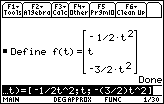Show that, for arbitrary t1, t2, t3, the triple scalar product of f(t1), f(t2), f(t3) equals zero (i.e. f(t1), f(t2), f(t3) are coplanar):

1) Press [CATALOG] [C] and locate crossP.2) Press [(] [F] [(] [T]  [)] [,].
3) Press [F] [(] [T]  [)] [)] [ENTER].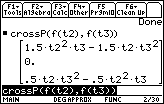4) Press [CATALOG] [D] and locate dotP.5) Press [F] [(] [T]  [)] [,].
6) Press [2ND] [ANS] [)] [ENTER].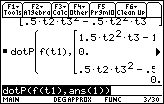Please Note: The answer is zero. Since t1, t2, t3 were arbitrary, one may conclude that the points corresponding to the tip of the vector for any value of t all lie in the same plane.

Now determine the plane in which the curve lies. The unit tangent vector, tt(t), and the principal unit normal vector, n(t), for this curve lie within this plane:

Define tt(t) = d(f(t), t)/norm(d(f(t), t)) (Here, d is the derivative command.)

1) Press [CATALOG] [D] and locate Define.
2) Press [T] [T] [(] [T] [)] [=].
3) Press [2ND] [d] [F] [(] [T] [)] [/].
4) Press [CATALOG] [N] and locate norm(.5) Press [2ND] [d] [F] [(] [T] [)] [,].
6) Press [T] [)] [)] [ENTER].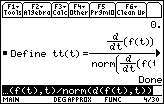Using the fact that the derivative of the arc length of the curve, s(t), is equal to norm(d(f(t),t)), use the formula.

n(t) =d(tt(t), t)/d(s(t), t) to define n(t).

Define: n(t) = d(tt(t), t)/norm(d(f(t), t))

1) Press [N] [(] [T] [)] [=].
2) Press [2ND] [d].
3) Press [T] [T] [(] [T] [)] [,] [T] [)] [/].
4) Press [CATALOG] and locate norm(.
5) Press [2ND] [d].
6) Press [F] [(] [T] [)] [,] [T] [)] [)] [ENTER].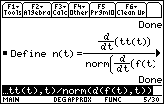The binormal vector, b(t), is orthogonal to the vectors tt(t) and n(t).

Define: b(t) = crossP(tt(t), n(t)):

1) Press [CATALOG] [D] and locate Define.
2) Press [B] [(] [T] [)] [=].
3) Press [CATALOG] [C] and locate crossP(.
4) Press [T] [T] [(] [T] [)] [,].
5) Press [N] [(] [T] [)] [)] [ENTER].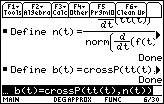It is now possible to transform the vector f(t) into the new coordinates.

An infinite number of unit vectors are now normal to the plane in which the curve lies. Only one of these is needed, along with a point on the curve (since the curve lies within the plane) to determine the equation of the plane. For simplicity, choose f(0) and b(0):

1) Press [F] [(]  [)] [ENTER].
2) Press [B] [(]  [)] [ENTER].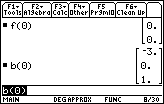We now see that the equation for the desired plane is -(6)*x + z = 0, or z = (6)*x.

Returning to the original vector, f(t) = [-1/2*t2; t; -(3/2)*t2], which we can write as f(t) = [x(t), y(t), z(t)], where:

• x(t) = -1/2*t2
• y(t) = t
• z(t) = -(3/2)*t2

Now solve for t in terms of y, so that:

• x = -1/2*y2
• z = -(3/2)*y2

If a new coordinate zz is defined on the plane in which the curve lies, i.e. zz = z = (6)*x, in the new coordinate system in the plane:

zz = -1/2*y2

This can be graphed using standard function graphing (setting y1 = -1/2*x2):

1) Press [◊] [Y=].
2) Press [(-)]  [/]  [x].
3) Press [X] [^]  [ENTER].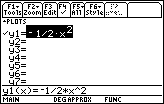4) Press [◊] [GRAPH].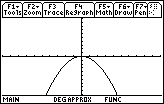Please see the TI-89 family, TI-92 family and Voyage 200 guidebooks for additional information.